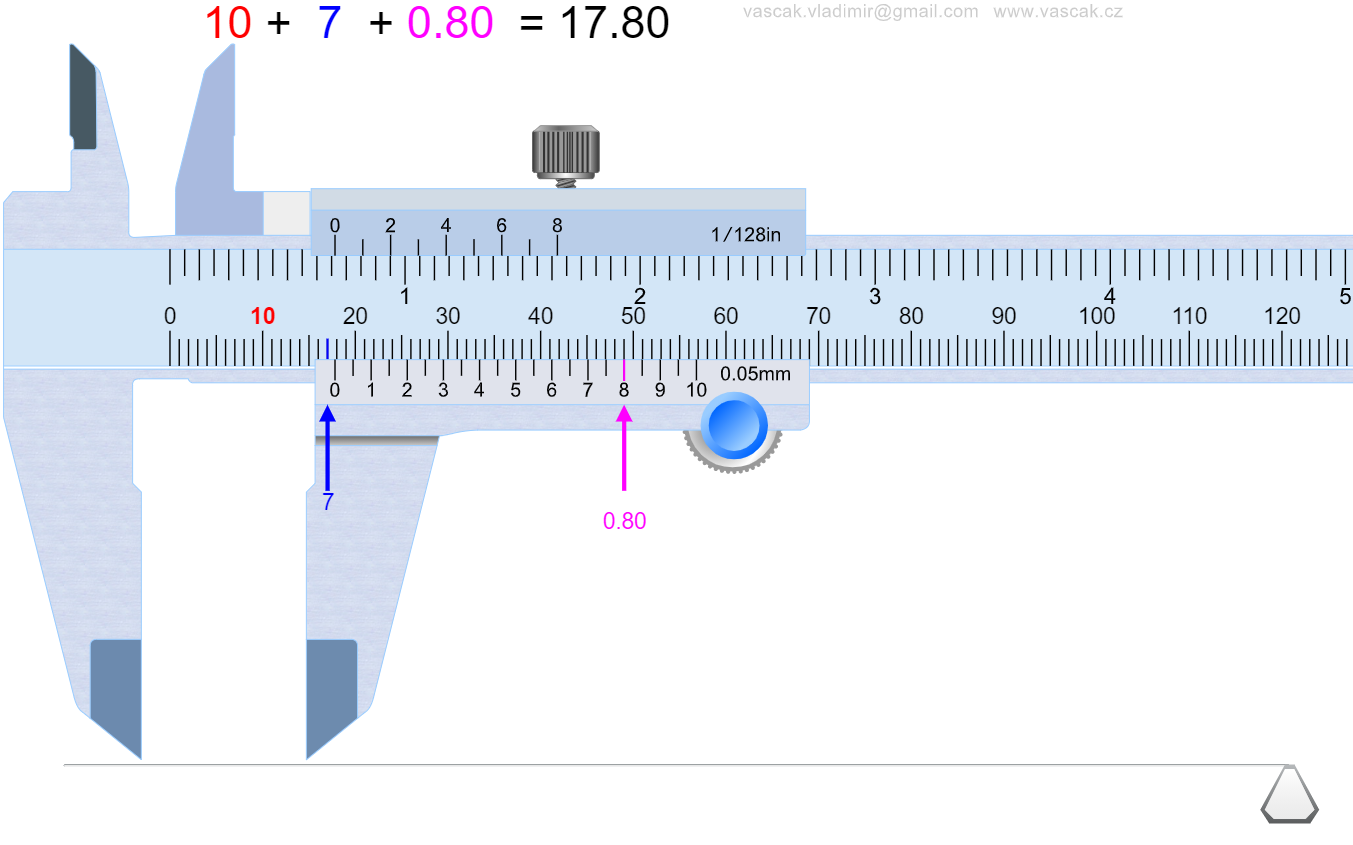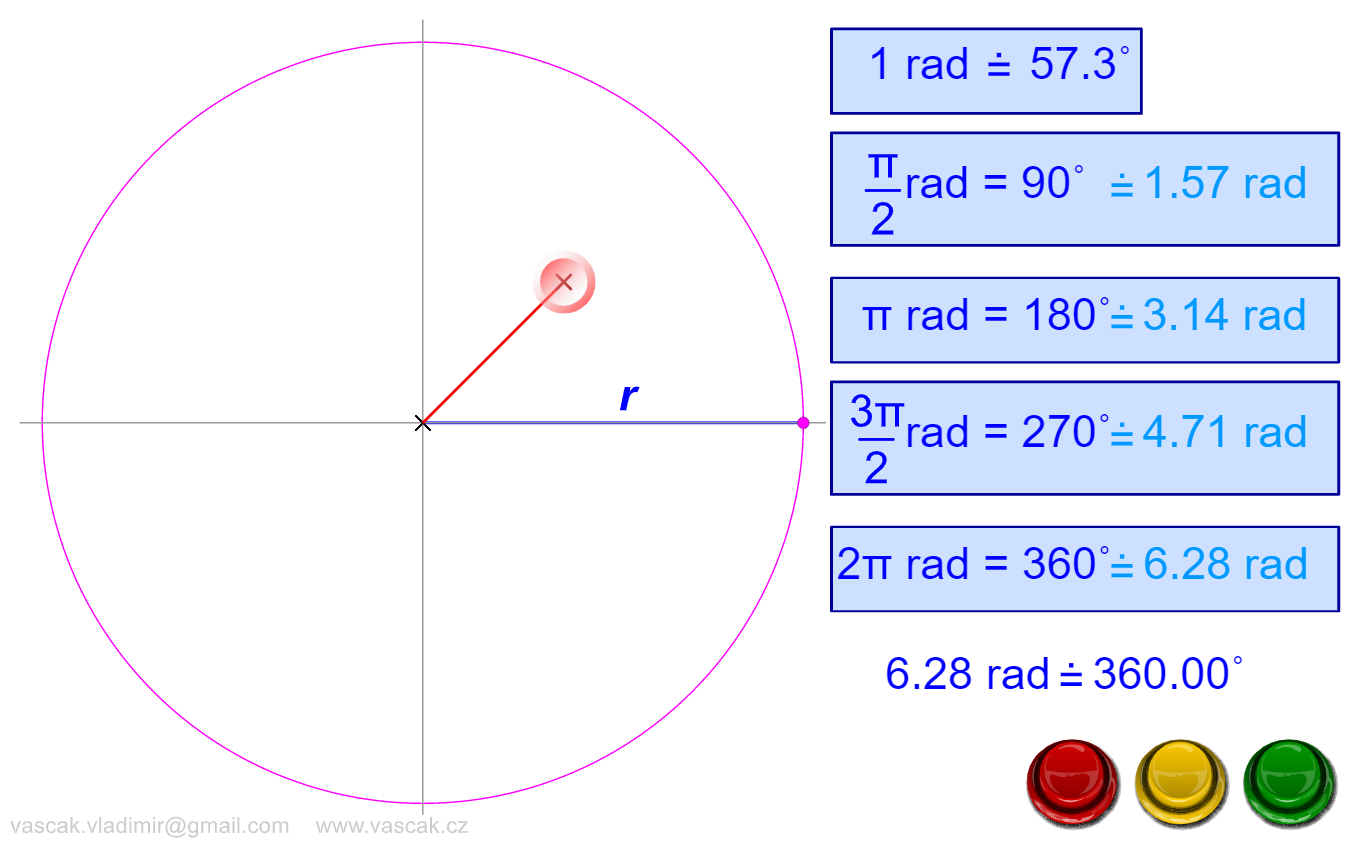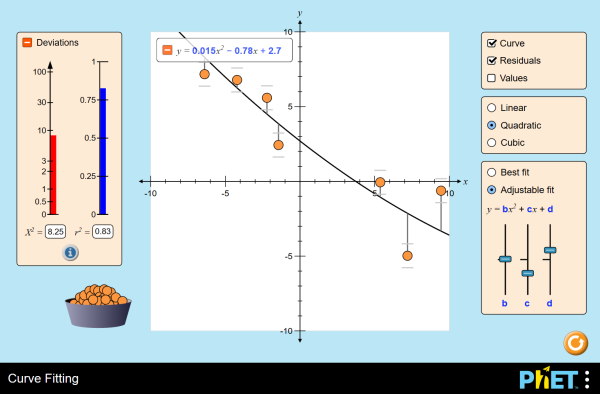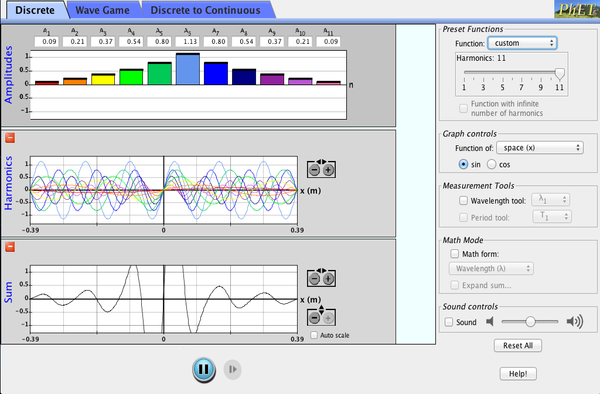# Mathematical Concepts and Skills

Student Learning Objectives
Lessons / Lecture Notes
Important Equations
Example Problems
Applets and Animations

Student Learning Objectives

• To understand basic trigonometric and geometric relations.
• To understand how to convert from one set of units to another.
• To understand the proper use of significant figures.
• To gain initial experience with graphical addition and subtraction of vectors.
• To gain initial experience with displacement and velocity vectors

Lessons / Lecture Notes

PY105 Notes from Boston University (algebra-based):

HyperPhysics (calculus-based)

Physics 4A notes from Dr. Bobby W.S. Lau (calculus-based)

Important Equations (for algebra-based Physics)

Example Problems

Example Problems for algebra-based physics (from College Physics 2nd Edition by Knight, Jones, and Field):

Example Problems (Representing Motion) (See problems 6 - 9, 12, and 13)
Solutions to Example Problems (Representing Motion)

Example Problems for calculus-based physics (from Physics for Scientists and Engineers 4th Edition by Knight):

Example Problems (Concepts of Motion)
Solutions to Example Problems (Concepts of Motion)

Applets and Animations

 Powers of Ten View the Milky Way at 10 million light years from the Earth. Then move through space towards the Earth in successive orders of magnitude until you reach a tall oak tree just outside the buildings of the National High Magnetic Field Laboratory in Tallahassee, Florida. After that, begin to move from the actual size of a leaf into a microscopic world that reveals leaf cell walls, the cell nucleus, chromatin, DNA and finally, into the subatomic universe of electrons and protons. Vernier CalipersA very nice applet showing how to measure distances using a Vernier Caliper. RadiansLearn about how a radian is defined and the radian value of common angles. Curve FittingDrag data points and their error bars and watch the best-fit polynomial curve update instantly. You choose the type of fit: linear, quadratic, or cubic. The reduced chi-square statistic shows you when the fit is good. Or you can try to find the best fit by manually adjusting fit parameters. Fourier: Making WavesLearn how to make waves of all different shapes by adding up sines or cosines. Make waves in space and time and measure their wavelengths and periods. See how changing the amplitudes of different harmonics changes the waves. Compare different mathematical expressions for your waves. Plinko ProbabilityDrop balls through a triangular grid of pegs and see them accumulate in containers. Switch to a histogram view and compare the distribution of balls to an ideal binomial distribution. Adjust the binary probability and develop your knowledge of statistics!